Home | | Electronic Circuits I | Biasing of MOSFET

# Biasing of MOSFET

*N-channel enhancement mode MOSFET circuit shows the source terminal at ground potential and is common to both the input and output sides of the circuit.

Biasing of MOSFET

*N-channel enhancement mode MOSFET circuit shows the source terminal at ground potential and is common to both the input and output sides of the circuit. *The coupling capacitor acts as an open circuit to d.c. but it allows the signal voltage to be coupled to the gate of the MOSFETAs Ig = 0 in VG is given as,Assume VG > VT , MOSFET is biased in the saturation region, the drain current is,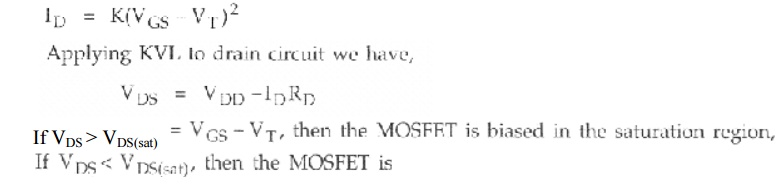Biased in the nonsaturation region, and the drain current is given by, ID

Example problem-1Here, the source is tied to +VDD, Which become signal ground in the a.c. equivalent circuit. Thus it is also a common-source circuit.

The d.c. analysis for this circuit is essentially the same as for the n-channel MOSFET circuit. The gate voltage is given by,Load Line and Modes of Operation

The load line gives a graphical picture showing the region in whichthe MOSFET is biased. Consider the common-source circuit shown in Fig. (a).

Writing Kirchhoff's voltage law around the drain-source loop results VDs = VDD -IDRD, which is the load line equation. It shows a linear relationship between the drain current and drain-to-source voltage. Fig. (b) shows the VDS(sat) characteristic for the MOSFETThe two end points of the load line are determine in the usual manner. If the drain current = 0, then VDS= 10 v; if VDS = 0, then drain current = 10/40 = 0.25 mA. The Q-point of the MOSFET is given by the d.c. drain current (ID) and drain-to-source voltage (VDS) and it is always on the load line, as shown in the Fig. b).

If the gate-to-source voltage is less than V1, the drain current is zero and the MOSFET is in cut-off. As the gate-to- source voltage becomes just greater than the threshold voltage, the MOSFET turns ON and is biased in the saturation region. As V GS increases, the Q-point moves up the load line. The transition point is the boundary between the saturation and non-saturation regions. It is the point where,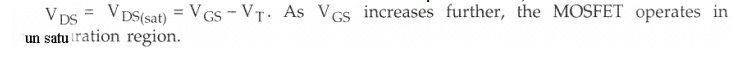Common Source circuit for EMOSFET with source resistorVoltage Divider BiasBiasing Circuit for D MOSFET

Biasing circuits for depletion type MOSFET are quite similar to the circuits used for JFET biasing. The primary difference between the two is the fact that depletion type MOSFETs also permit operating points with positive value of V6s for n-channel and negative values of V6s for p-channel MOSFET. To have positive value of V GS for n-channel and negative value of V6s for p-channel self bias circuit is unsuitable.

Example problem-1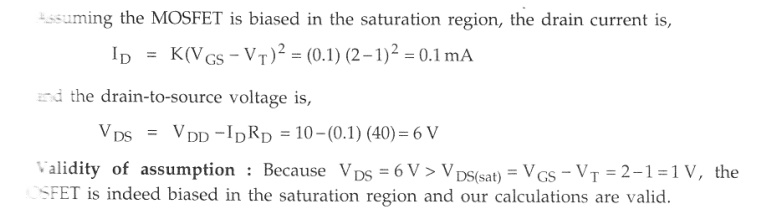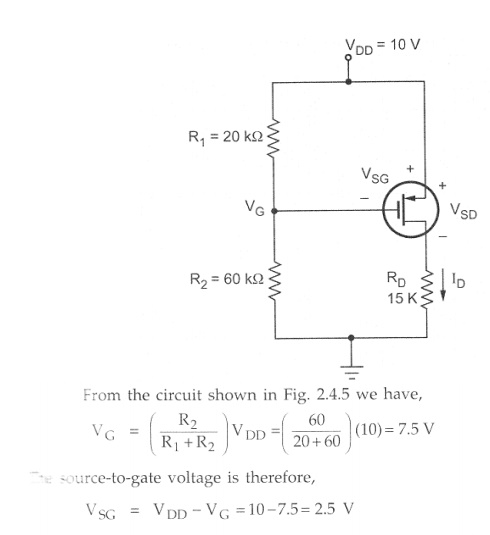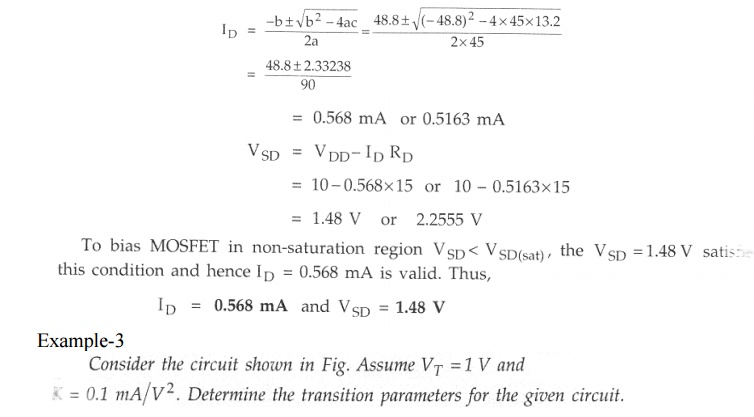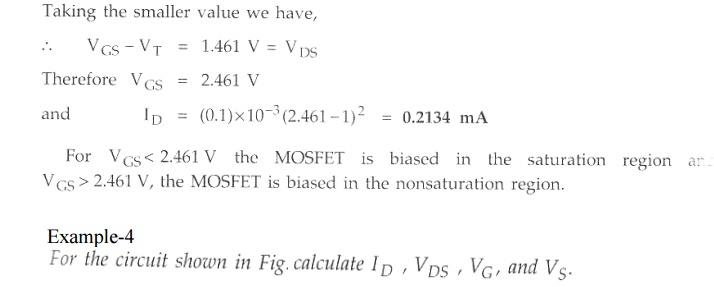Study Material, Lecturing Notes, Assignment, Reference, Wiki description explanation, brief detail
Electronic Circuits : Biasing of Discrete BJT and MOSFET : Biasing of MOSFET |